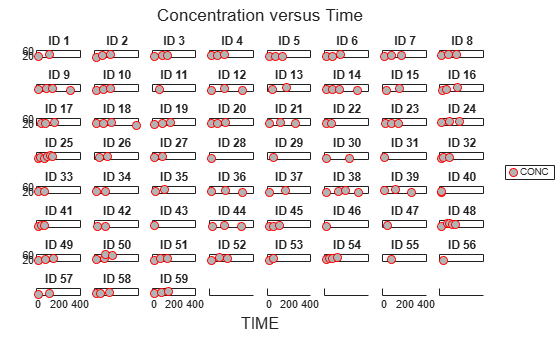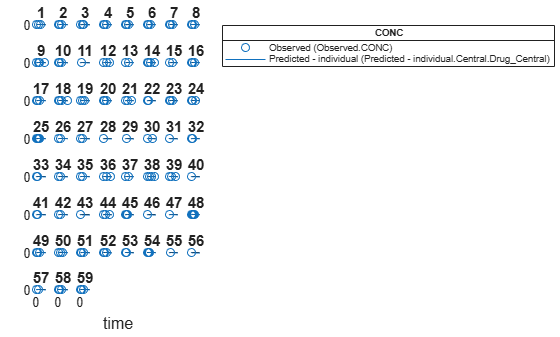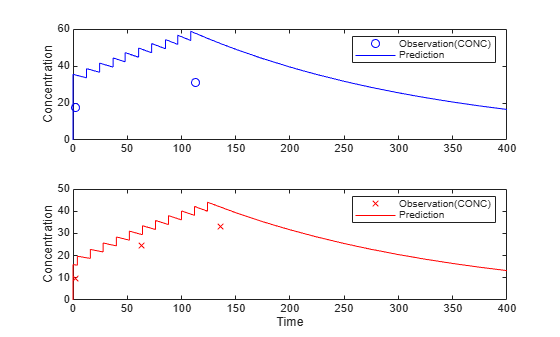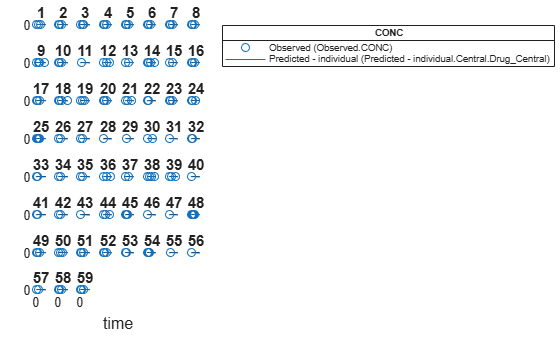# predict

Simulate and evaluate fitted SimBiology model

## Syntax

``````[ynew,parameterEstimates] = predict(resultsObj)``````
``````[ynew,parameterEstimates] = predict(resultsObj,data,dosing)``````
``````[ynew,parameterEstimates] = predict(___,Name,Value)``````

## Description

``````[ynew,parameterEstimates] = predict(resultsObj)``` returns simulation results `ynew` from evaluating the fitted SimBiology® model. The estimated parameter values `parameterEstimates` used to compute `ynew` are from the original fit by `sbiofitmixed`.```
``````[ynew,parameterEstimates] = predict(resultsObj,data,dosing)``` returns simulation results `ynew` from evaluating the fitted SimBiology model by using the specified `data` and `dosing` information.```

example

``````[ynew,parameterEstimates] = predict(___,Name,Value)``` uses additional options specified by one or more name-value arguments. TipUse this method to get model responses at specific time points or to predict model responses using different covariate data and dosing information. ```

## Examples

collapse all

Estimate nonlinear mixed-effects parameters using clinical pharmacokinetic data collected from 59 infants. Evaluate the fitted model given new data or dosing information.

This example uses data collected on 59 preterm infants given phenobarbital during the first 16 days after birth . `ds` is a table containing the concentration-time profile data and covariate information for each infant (or group).

`load pheno.mat ds`

Convert to groupedData

Convert the data to the groupedData format for parameter estimation.

`data = groupedData(ds);`

Display the first few rows of `data`.

`data(1:5,:)`
```ans = 5x6 groupedData ID TIME DOSE WEIGHT APGAR CONC __ ____ ____ ______ _____ ____ 1 0 25 1.4 7 NaN 1 2 NaN 1.4 7 17.3 1 12.5 3.5 1.4 7 NaN 1 24.5 3.5 1.4 7 NaN 1 37 3.5 1.4 7 NaN ```

Visualize Data

Display the data in a trellis plot.

```t = sbiotrellis(data, 'ID', 'TIME', 'CONC', 'marker', 'o',... 'markerfacecolor', [.7 .7 .7], 'markeredgecolor', 'r', ... 'linestyle', 'none'); t.plottitle = 'Concentration versus Time';```Create a One-Compartment PK Model

Create a simple one-compartment PK model, with bolus dose administration and linear clearance elimination, to fit the data.

```pkmd = PKModelDesign; addCompartment(pkmd,'Central','DosingType','Bolus',... 'EliminationType','linear-clearance',... 'HasResponseVariable',true,'HasLag',false); onecomp = pkmd.construct;```

Map model species to response data.

`responseMap = 'Drug_Central = CONC';`

Define Estimated Parameters

The parameters to estimate in this model are the volume of the central compartment (`Central`) and the clearance rate (`Cl_Central`). `sbiofitmixed` calculates fixed and random effects for each parameter. The underlying algorithm computes normally distributed random effects, which might violate constraints for biological parameters that are always positive, such as volume and clearance. Therefore, specify a transform for the estimated parameters so that the transformed parameters follow a normal distribution. The resulting model is

`$log\left({V}_{i}\right)=log\left({\varphi }_{V,i}\right)={\theta }_{V}+{\eta }_{V,i}$`

and

`$log\left(C{l}_{i}\right)=log\left({\varphi }_{Cl,i}\right)={\theta }_{Cl}+{\eta }_{Cl,i},$`

where $\theta$, $eta$, and $\varphi$ are the fixed effects, random effects, and estimated parameter values respectively, calculated for each infant (group) $i$. Some arbitrary initial estimates for V (volume of central compartment) and Cl (clearance rate) are used here in the absence of better empirical data.

`estimatedParams = estimatedInfo({'log(Central)','log(Cl_Central)'},'InitialValue',[1 1]);`

Define Dosing

All infants were given the drug, represented by the `Drug_Central` species, where the dosing schedule varies among infants. The amount of drug is listed in the data variable DOSE. You can automatically generate dose objects from the data and use them during fitting. In this example, `Drug_Central` is the target species that receives the dose.

```sampleDose = sbiodose('sample','TargetName','Drug_Central'); doses = createDoses(data,'DOSE','',sampleDose);```

Fit the Model

Use `sbiofitmixed` to fit the one-compartment model to the data.

`nlmeResults = sbiofitmixed(onecomp,data,responseMap,estimatedParams,doses,'nlmefit');`

Visualize Results

Visualize the fitted results using individual-specific parameter estimates.

`plot(nlmeResults,'ParameterType','individual');`Use New Dosing Data to Simulate the Fitted Model

Suppose you want to predict how infants 1 and 2 would have responded under different dosing amounts. You can predict their responses as follows.

Create new dose objects with new dose amounts.

```dose1 = doses(1); dose1.Amount = dose1.Amount*2; dose2 = doses(2); dose2.Amount = dose2.Amount*1.5;```

Use the `predict` function to evaluate the fitted model using the new dosing data. If you want response predictions at particular times, provide the new output time vector. Use the 'ParameterType' option to specify individual or population parameters to use. By default, `predict` uses the population parameters when you specify output times.

```timeVec = [0:25:400]; newResults = predict(nlmeResults,timeVec,[dose1;dose2],'ParameterType','population');```

Visualize the predicted responses while overlapping the experimental data for infants 1 and 2.

```figure; subplot(2,1,1) plot(data.TIME(data.ID == 1),data.CONC(data.ID == 1),'bo') hold on plot(newResults(1).Time,newResults(1).Data,'b') hold off ylabel('Concentration') legend('Observation(CONC)','Prediction') subplot(2,1,2) plot(data.TIME(data.ID == 2),data.CONC(data.ID == 2),'rx') hold on plot(newResults(2).Time,newResults(2).Data,'r') hold off legend('Observation(CONC)','Prediction') ylabel('Concentration') xlabel('Time')```Create a Covariate Model for the Covariate Dependencies

Suppose there is a correlation between volume and weight, and possibly volume and APGAR score. Consider the effect of weight by modeling two of these covariate dependencies: the volume of central (`Central`) and the clearance rate (`Cl_Central`) vary with weight. The model becomes

`$log\left({V}_{i}\right)=log\left({\varphi }_{V,i}\right)={\theta }_{V}+{\theta }_{V/weight}*weigh{t}_{i}+{\eta }_{V,i}$`

and

`$log\left(C{l}_{i}\right)=log\left({\varphi }_{Cl,i}\right)={\theta }_{Cl}+{\theta }_{Cl/weight}*weigh{t}_{i}+{\eta }_{Cl,i}$`

Use the `CovariateModel` object to define the covariate dependencies. For details, see Specify a Covariate Model.

```covModel = CovariateModel; covModel.Expression = ({'Central = exp(theta1 + theta2*WEIGHT + eta1)',... 'Cl_Central = exp(theta3 + theta4*WEIGHT + eta2)'});```

Use `constructDefaultInitialEstimate` to create an `initialEstimates` struct.

`initialEstimates = covModel.constructDefaultFixedEffectValues;`

Use the `FixedEffectNames` property to display the thetas (fixed effects) defined in the model.

`covModel.FixedEffectNames`
```ans = 4x1 cell {'theta1'} {'theta3'} {'theta2'} {'theta4'} ```

Use the `FixedEffectDescription` property to show the descriptions of corresponding fixed effects (thetas) used in the covariate expression. For example, `theta2` is the fixed effect for the weight covariate that correlates with the volume (`Central`), denoted as 'Central/WEIGHT'.

`disp('Fixed Effects Description:');`
```Fixed Effects Description: ```
`disp(covModel.FixedEffectDescription);`
``` {'Central' } {'Cl_Central' } {'Central/WEIGHT' } {'Cl_Central/WEIGHT'} ```

Set the initial guesses for the fixed-effect parameter values for `Central` and `Cl_Central` using the values estimated from fitting the base model.

```initialEstimates.theta1 = nlmeResults.FixedEffects.Estimate(1); initialEstimates.theta3 = nlmeResults.FixedEffects.Estimate(2); covModel.FixedEffectValues = initialEstimates;```

Fit the Model

`nlmeResults_cov = sbiofitmixed(onecomp,data,responseMap,covModel,doses,'nlmefit');`

Display Fitted Parameters and Covariances

`disp('Estimated Fixed Effects:');`
```Estimated Fixed Effects: ```
`disp(nlmeResults_cov.FixedEffects);`
``` Name Description Estimate StandardError __________ _____________________ ________ _____________ {'theta1'} {'Central' } -0.45664 0.078933 {'theta3'} {'Cl_Central' } -5.9519 0.1177 {'theta2'} {'Central/WEIGHT' } 0.52948 0.047342 {'theta4'} {'Cl_Central/WEIGHT'} 0.61954 0.071386 ```
`disp('Estimated Covariance Matrix:');`
```Estimated Covariance Matrix: ```
`disp(nlmeResults_cov.RandomEffectCovarianceMatrix);`
``` eta1 eta2 ________ ________ eta1 0.046503 0 eta2 0 0.041609 ```

Visualize Results

Visualize the fitted results using individual-specific parameter estimates.

`plot(nlmeResults_cov,'ParameterType','individual');`Use New Covariate Data to Evaluate the Fitted Model

Suppose you want to explore the responses of infants 1 and 2 using different covariate data, namely `WEIGHT`. You can do this by specifying the new `WEIGHT` data. The `ID` variable of the data corresponds to individual infants.

```newData = data(data.ID == 1 | data.ID == 2,:); newData.WEIGHT(newData.ID == 1) = 1.3; newData.WEIGHT(newData.ID == 2) = 1.4;```

Simulate the responses of infants 1 and 2 using the new covariate data.

`[newResults_cov, newEstimates] = predict(nlmeResults_cov,newData,[dose1;dose2]);`

`newEstimates` contains the updated parameter estimates for each individual (infants 1 and 2) after the model is reevaluated using the new covariate data.

`newEstimates`
```newEstimates=4×3 table Group Name Estimate _____ ______________ _________ 1 {'Central' } 2.5596 1 {'Cl_Central'} 0.0065965 2 {'Central' } 1.7123 2 {'Cl_Central'} 0.0064806 ```

Compare to the estimated values from the original fit using the old covariate data.

```nlmeResults_cov.IndividualParameterEstimates( ... nlmeResults_cov.IndividualParameterEstimates.Group == '1' | ... nlmeResults_cov.IndividualParameterEstimates.Group == '2',:)```
```ans=4×3 table Group Name Estimate _____ ______________ _________ 1 {'Central' } 2.6988 1 {'Cl_Central'} 0.0070181 2 {'Central' } 1.8054 2 {'Cl_Central'} 0.0068948 ```

Visualize the new simulation results together with the experimental data for infant 1 and 2.

```figure; subplot(2,1,1); plot(data.TIME(data.ID == 1),data.CONC(data.ID == 1),'bo') hold on plot(newResults_cov(1).Time,newResults_cov(1).Data,'b') hold off ylabel('Concentration') legend('Observation(CONC)','Prediction','Location','NorthEastOutside') subplot(2,1,2) plot(data.TIME(data.ID == 2),data.CONC(data.ID == 2),'rx') hold on plot(newResults_cov(2).Time,newResults_cov(2).Data,'r') hold off legend('Observation(CONC)','Prediction','Location','NorthEastOutside') ylabel('Concentration') xlabel('Time')```References

 Grasela, T. H. Jr., and S. M. Donn. "Neonatal population pharmacokinetics of phenobarbital derived from routine clinical data." Dev Pharmacol Ther 1985:8(6). 374-83.

## Input Arguments

collapse all

Estimation results, specified as a scalar `NLMEResults object`, which contains nonlinear mixed-effects estimation results returned by `sbiofitmixed`.

Grouped data or output times, specified as a `groupedData object`, vector, or cell array of vectors of output times.

If `data` is a `groupedData` object, it must have both group labels and output times specified. The group labels can refer to new groups or existing groups from the original fit. If the mixed-effects model from the original fit (returned by `sbiofitmixed`) uses covariates, the `groupedData` object must also contain the covariate data with the same labels for the covariates (`CovariateLabels` property) specified in the original `CovariateModel` object.

By default, individual parameter estimates are used for simulating groups from the original fit, while population parameters are used for new groups, if any. See the `value` argument description for details.

The total number of simulation results in the output `ynew` depends on the number of groups or output time vectors in `data` and the number of rows in the `dosing` matrix. For details, see the table in the `ynew` argument description.

Dosing information, specified as empty `[]` or `{}`, 2-D matrix or cell vector of SimBiology dose objects (`ScheduleDose object` or `RepeatDose object`).

If `dosing` is empty, no doses are applied during simulation, even if the model has active doses.

For a matrix of dose objects, it must have a single row or one row per group in the input data. If it has a single row, the same doses are applied to all groups during simulation. If it has multiple rows, each row is applied to a separate group, in the same order as the groups appear in the input data. Multiple columns are allowed so that you can apply multiple dose objects to each group.

For a cell vector of doses, it must have one element or one element per group in the input data. Each element must be `[]` or a vector of doses. Each element of the cell is applied to a separate group, in the same order as the groups appear in the input data.

In addition to manually constructing dose objects using `sbiodose`, if the input `groupedData` object has dosing information, you can use the `createDoses` method to construct doses.

Dose objects of the `dosing` input must be consistent with the original dosing data used with `sbiofitmixed`. The objects must have the same values for dose properties (such as `TargetName`) or must be parameterized in the same way as the original dosing data. For instance, suppose that the original dosing matrix has two columns of doses, where the doses in the first column target species x and those in the second column target species y. Then `dosing` must have doses in the first column targeting species x and those in the second column targeting species y. A parameterized dose example is as follows. Suppose that the `Amount` property of a dose used in the original `sbiofitmixed` call is parameterized to a model-scoped parameter `'A'`. All doses for the corresponding group (column) in the `dosing` matrix input must have the `Amount` property parameterized to `'A'`.

The number of rows in the `dosing` matrix or number of elements in the `dosing` cell vector and the number of groups or output time vectors in `data` determine the total number of simulation results in the output `ynew`. For details, see the table in the `ynew` argument description.

Note

If `UnitConversion` is turned on for the underlying SimBiology model that was used for fitting, `dosing` must specify valid amount and time units.

### Name-Value Arguments

Specify optional pairs of arguments as `Name1=Value1,...,NameN=ValueN`, where `Name` is the argument name and `Value` is the corresponding value. Name-value arguments must appear after other arguments, but the order of the pairs does not matter.

Before R2021a, use commas to separate each name and value, and enclose `Name` in quotes.

Example: `'ParameterType','population'` specifies to use population parameter estimates.

Parameter type, specified as `'individual'` (default) or `'population'`. If `value` is `'population'`, the `predict` method returns the simulation results using the population parameter estimates, that is, parameter values that are estimated using fixed effects (θs) only. The estimated parameter values used in simulation are identical to those in the `resultsObj.PopulationParameterEstimates` property, unless you specify a new `groupedData` object `data` with new covariate data. In this case, the method reevaluates the covariate model and the parameter estimates based on the new `groupedData` and covariate data.

If `value` is `'individual'`, the method returns the simulation results using the corresponding parameter values of the group in the `resultsObj.IndividualParameterEstimates` property. These values include both fixed- and random-effects estimates, that is, parameter values estimated using both fixed effects (θs) and random effects (ηs). If `data` contains new groups, only fixed effects (population parameter estimates of the results object) are used for these groups.

By default, `predict` uses the individual parameter estimates of the results object when `data` is a `groupedData` object. If `data` is a vector of output times or cell array of vectors, `predict` uses the population parameter estimates of the results object instead.

Data Types: `char` | `string`

Variants to apply, specified as an empty array (`[]`, `{}`), 2-D matrix or cell vector of variant objects.

If you do not specify this argument, the function has the following behavior depending on whether the second input argument (`data`) is specified also or not.

• If `data` is not specified, the function applies the group-specific variants from the original call to `sbiofitmixed`.

• If `data` is a vector or cell array of output times, the function does not apply the group-specific variants.

• If `data` is a `groupedData` object, the function applies variants only to groups whose group identifier matches a group identifier in the original training data that was used in the call to `sbiofitmixed`.

Note

• The baseline variants that were specified by the variants positional input argument in the original call to `sbiofitmixed` are always applied to the model, and they are applied before any group-specific variants.

• If there are no baseline variants, that is, you did not specify the `variants` input when calling `sbiofitmixed`, the function still applies the model active variants if there are any.

If the argument value is `[]` or `{}`, the function applies no group-specific variants.

If it is a matrix of variants, it must have either one row or one row per group. Each row is applied to a separate group, in the same order as the groups appear in `data` or `dosing`. If it has a single row, the same variants are applied to all groups during simulation. If there are multiple columns, the variants are applied in order from the first column to the last.

If it is a cell vector of variant objects, the number of cells must be one or must match the number of groups in the input data. Each element must be `[]` or a vector of variants. If there is a single cell containing a vector of variants, they are applied to all simulations. If there are multiple cells, the variants in the ith cell are applied to the simulation of the ith group.

The function defines the number of groups by examining the `data`, and `dosing` input arguments.

• `data` can have `1` or N groups.

• If `data` and `dosing` arguments are not specified, then the default data and dosing are determined as follows:

• For unpooled fits, they are the data and dosing for the single group associated with that fit results.

• For all other fits, they are the entire set of data and dosing associated with the call to `sbiofitmixed`.

## Output Arguments

collapse all

Simulation results, returned as a vector of `SimData` objects. The states reported in `ynew` are the states included in the `responseMap` input argument of `sbiofitmixed` and any other states listed in the `StatesToLog` property of the runtime options (`RuntimeOptions`) of the SimBiology model.

The total number of simulation results in `ynew` depends on the number of groups or output time vectors in `data` and the number of rows in the `dosing` matrix.

Number of groups or output time vectors in `data`Number of rows in the `dosing` matrixSimulation results

`1`

`0`, that is, `dosing` is empty `[]`

The total number of `SimData` objects in `ynew` is 1.

No doses are applied during simulation.

`1`

`1`

The total number of `SimData` objects in `ynew` is 1.

The given row of doses is applied during the simulation.

`1`

N

The total number of `SimData` objects in `ynew` is N.

Each row of `dosing` is applied to each simulation.

N

`0`, that is, `dosing` is empty `[]`

The total number of `SimData` objects in `ynew` is N.

No doses are applied during simulation.

N

`1`

The total number of `SimData` objects in `ynew` is N.

The same row of doses is applied to each simulation.

NN

The total number of `SimData` objects in `ynew` is N.

Each row of `dosing` is applied to a separate group, in the same order that groups appear in `data`.

MNThe function throws an error when MN.

Estimated parameter values used for the predicted simulation results, returned as a table.

If `'ParameterType'` is `'individual'`, the reported parameter values are identical to the values in the `resultsObj.IndividualParameterEstimates` property. However, if `data` contains new groups, then only population parameter estimates (fixed effects) are used for these groups. The corresponding reported values in `parameterEstimates` for these groups are identical to the values in `resultsObj.PopulationParameterEstimates`.

If `'ParameterType'` is `'population'`, the reported parameter values are identical to the values in the `resultsObj.PopulationParameterEstimates` property unless you specify new covariate information in `data`. See the `value` argument description for details.

If `data` is a vector or a cell array of vectors of output times, the reported parameter values are identical to the values in `resultsObj.PopulationParameterEstimates`. Also, the groups reported represent the enumeration of simulations performed and are unrelated to group names in the original fit.

## Version History

Introduced in R2014a